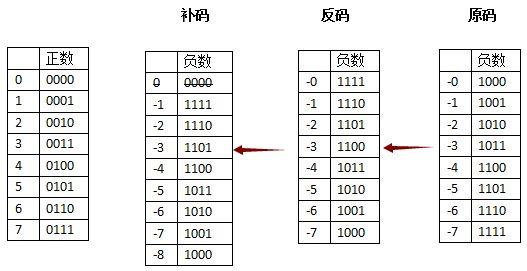### 存储数字

37 >> 100101
-37 >> -100101 // 计算机只能存 0 1，所以存的是 2 的补码后的数
0.75 >> 0.11 // 浮点数同理也是计算后的

### 关于原码，反码，补码### 存储字符

ASCII 码有 128 个，对应 0 - 128, 一个字节就可表示

### 存储汉字

GBK 有 6000 多个汉字，可以用两个字节表示

### Unicode

Unicode 本来用 4 个字节表示

### es5 的问题

js 使用了 UCS-2 的编码方式，只能表示两个字节的字符，es6 解决了这个问题，所以 `for (let a of string)` 就没问题了

### 一些想法

• 看完这个，突然对文件大小有了更加清晰的看法，想起了以前的一个题

function getSize(size) {
if (!size) {
return ‘’
}

``````var n = 1024

if (size < n) {
return size + 'B'
} else if (size < Math.pow(n, 2)) {
return Math.floor(size / n) + 'KB'
} else if (size < Math.pow(n, 3)) {
return Math.floor(size / Math.pow(n, 2)) + 'MB'
} else if (size < Math.pow(n, 4)) {
return Math.floor(size / Math.pow(n, 3)) + 'GB'
} else {
return Math.floor(size / Math.pow(n, 4)) + 'TB'
}
``````

}